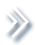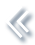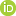####ArchivesReview Articles

## Optimal mean-variance reinsurance and investment strategy with constraints in a non-Markovian regime-switching model

Liming Zhang ,

Key Laboratory of Advanced Theory and Application in Statistics and Data Science-MOE, School of Statistics, East China Normal University, Shanghai, Peoples Republic of ChinaRongming Wang ,

Key Laboratory of Advanced Theory and Application in Statistics and Data Science-MOE, School of Statistics, East China Normal University, Shanghai, Peoples Republic of ChinaJiaqin Wei

Key Laboratory of Advanced Theory and Application in Statistics and Data Science-MOE, School of Statistics, East China Normal University, Shanghai, Peoples Republic of Chinajqwei@stat.ecnu.edu.cn

Pages 214-227 | Received 03 Jun. 2019, Accepted 25 Nov. 2019, Published online: 30 Jan. 2020,
• Abstract
• References
• Citations

Abstract

This paper is devoted to study the proportional reinsurance/new business and investment problem under the mean-variance criterion in a continuous-time setting. The strategies are constrained in the non-negative cone and all coefficients in the model except the interest rate are stochastic processes adapted the filtration generated by a Markov chain. With the help of a backward stochastic differential equation driven by the Markov chain, we obtain the optimal strategy and optimal cost explicitly under this non-Markovian regime-switching model. The cases with one risky asset and Markov regime-switching model are considered as special cases.

## References

1. Bai, L., & Zhang, H. (2008). Dynamic mean-variance problem with constrained risk control for the insurers. Mathematical Methods of Operations Research68(1), 181–205. doi: 10.1007/s00186-007-0195-4 [Crossref][Web of Science ®], [Google Scholar]
2. Bäuerle, N. (2005). Benchmark and mean-variance problems for insurers. Mathematical Methods of Operations Research62(1), 159–165. doi: 10.1007/s00186-005-0446-1 [Crossref][Web of Science ®], [Google Scholar]
3. Bi, J., & Guo, J. (2013). Optimal mean-variance problem with constrained controls in a jump-diffusion financial market for an insurer. Journal of Optimization Theory and Applications157(1), 252–275. doi: 10.1007/s10957-012-0138-y [Crossref][Web of Science ®], [Google Scholar]
4. Bi, J., Meng, Q., & Zhang, Y. (2014). Dynamic mean-variance and optimal reinsurance problems under the no-bankruptcy constraint for an insurer. Annals of Operations Research212(1), 43–59. doi: 10.1007/s10479-013-1338-z [Crossref][Web of Science ®], [Google Scholar]
5. Browne, S. (1995). Optimal investment policies for a firm with a random risk process: Exponential utility and minimizing the probability of ruin. Mathematics of Operations Research20(4), 937–958. doi: 10.1287/moor.20.4.937 [Crossref][Web of Science ®], [Google Scholar]
6. Chen, S., Li, Z., & Li, K. (2010). Optimal investment–reinsurance policy for an insurance company with var constraint. Insurance: Mathematics and Economics47(2), 144–153. [Crossref][Web of Science ®], [Google Scholar]
7. Chen, P., & Yam, S. C. P. (2013). Optimal proportional reinsurance and investment with regime-switching for mean–variance insurers. Insurance: Mathematics and Economics53(3), 871–883. [Crossref][Web of Science ®], [Google Scholar]
8. Cohen, S. N., & Elliott, R. J. (2008). Solutions of backward stochastic differential equations on Markov chains. Communications on Stochastic Analysis2(2), 251–262. doi: 10.31390/cosa.2.2.05 [Crossref], [Google Scholar]
9. Cohen, S. N., & Elliott, R. J. (2010). Comparisons for backward stochastic differential equations on Markov chains and related no-arbitrage conditions. The Annals of Applied Probability20(1), 267–311. doi: 10.1214/09-AAP619 [Crossref][Web of Science ®], [Google Scholar]
10. Cohen, S. N., & Szpruch, L. (2012). On Markovian solutions to Markov chain bsdes. Numerical Algebra, Control and Optimization2(2), 257–269. doi: 10.3934/naco.2012.2.257 [Crossref], [Google Scholar]
11. Elliott, R. J., Aggoun, L., & Moore, J. B. (2008). Hidden Markov models: Estimation and control. New York, NY: Springer. [Google Scholar]
12. Hu, Y., & Zhou, X. Y. (2005). Constrained stochastic LQ control with random coefficients, and application to portfolio selection. SIAM Journal on Control and Optimization44(2), 444–466. doi: 10.1137/S0363012904441969 [Crossref][Web of Science ®], [Google Scholar]
13. Li, D., & Ng, W. L. (2000). Optimal dynamic portfolio selection: Multiperiod mean-variance formulation. Mathematical Finance10(3), 387–406. doi: 10.1111/1467-9965.00100 [Crossref][Web of Science ®], [Google Scholar]
14. Liang, Z., & Bayraktar, E. (2014). Optimal reinsurance and investment with unobservable claim size and intensity. Insurance: Mathematics and Economics55, 156–166. [Crossref][Web of Science ®], [Google Scholar]
15. Markowitz, H. (1952). Portfolio selection. The Journal of Finance7(1), 77–91. [Crossref][Web of Science ®], [Google Scholar]
16. Promislow, S. D., & Young, V. R. (2005). Minimizing the probability of ruin when claims follow Brownian motion with drift. North American Actuarial Journal9(3), 110–128. doi: 10.1080/10920277.2005.10596214 [Taylor & Francis Online], [Google Scholar]
17. Shen, Y., Wei, J., & Zhao, Q. (2018). Mean–variance asset–liability management problem under non-Markovian regime-switching models. Applied Mathematics and Optimization. doi:10.1007/s00245-018-9523-8[Crossref][Web of Science ®], [Google Scholar]
18. Shen, Y., & Zeng, Y. (2015). Optimal investment–reinsurance strategy for mean–variance insurers with square-root factor process. Insurance: Mathematics and Economics62, 118–137. [Crossref][Web of Science ®], [Google Scholar]
19. Siu, T. K., & Shen, Y. (2017). Risk-minimizing pricing and esscher transform in a general non-Markovian regime-switching jump-diffusion model. Discrete and Continuous Dynamical Systems-series B22(7), 2595–2626. doi: 10.3934/dcdsb.2017100 [Crossref][Web of Science ®], [Google Scholar]
20. Walter, W. (1998). Ordinary differential equations. New York, NY:Springer-Verlag. [Crossref], [Google Scholar]
21. Wang, H., Wang, R., & Wei, J. (2019). Time-consistent investment-proportional reinsurance strategy with random coefficients for mean–variance insurers. Insurance: Mathematics and Economics85, 104–114. [Crossref][Web of Science ®], [Google Scholar]
22. Wang, T., & Wei, J. (2019). Mean–variance portfolio selection under a non-Markovian regime-switching model. Journal of Computational and Applied Mathematics350, 442–455. doi: 10.1016/j.cam.2018.10.040 [Crossref][Web of Science ®], [Google Scholar]
23. Xu, L., Zhang, L., & Yao, D. (2017). Optimal investment and reinsurance for an insurer under Markov-modulated financial market. Insurance: Mathematics and Economics74, 7–19. [Crossref][Web of Science ®], [Google Scholar]
24. Yang, H., & Zhang, L. (2005). Optimal investment for insurer with jump-diffusion risk process. Insurance: Mathematics and Economics37(3), 615–634. [Crossref][Web of Science ®], [Google Scholar]
25. Yi, B., Li, Z., Viens, F. G., & Zeng, Y. (2013). Robust optimal control for an insurer with reinsurance and investment under Heston's stochastic volatility model. Insurance: Mathematics and Economics53(3), 601–614. [Crossref][Web of Science ®], [Google Scholar]
26. Zeng, Y., Li, D., & Gu, A. (2016). Robust equilibrium reinsurance-investment strategy for a mean–variance insurer in a model with jumps. Insurance: Mathematics and Economics66, 138–152. [Crossref][Web of Science ®], [Google Scholar]
27. Zhang, X., & Siu, T. K. (2012). On optimal proportional reinsurance and investment in a Markovian regime-switching economy. Acta Mathematica Sinica, English Series28(1), 67–82. doi: 10.1007/s10114-012-9761-7 [Crossref][Web of Science ®], [Google Scholar]
28. Zhou, X. Y., & Li, D. (2000). Continuous-time mean-variance portfolio selection: A stochastic LQ framework. Applied Mathematics and Optimization42(1), 19–33. doi: 10.1007/s002450010003 [Crossref][Web of Science ®], [Google Scholar]
29. Zhou, X. Y., & Yin, G. (2003). Markowitz's mean-variance portfolio selection with regime switching: A continuous-time model. SIAM Journal on Control and Optimization42(4), 1466–1482. doi: 10.1137/S0363012902405583 [Crossref][Web of Science ®], [Google Scholar]